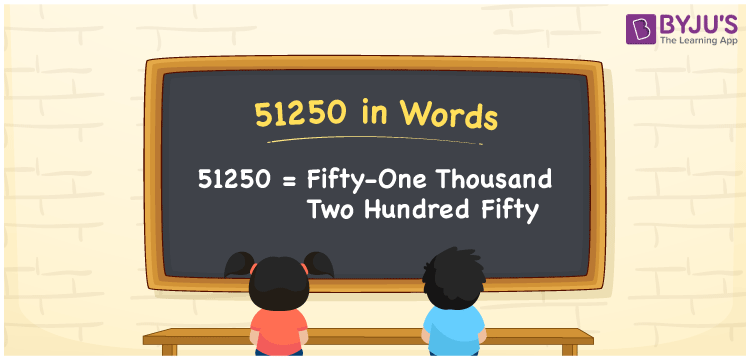# 51250 in Words

51250 in words can be written as Fifty-One Thousand Two Hundred Fifty. The concept of writing numbers in words can be learnt effectively in this article. If you buy a cupboard for Rs. 51250, then you can say that “I bought a cupboard for Fifty-One Thousand Two Hundred Fifty Rupees”. Learn in detail about the place value concept with the help of this article designed by experts at BYJU’S. So, 51250 can be read as “Fifty-One Thousand Two Hundred Fifty” in words.

 51250 in words Fifty-One Thousand Two Hundred Fifty Fifty-One Thousand Two Hundred Fifty in Numbers 51250

## 51250 in English Words## How to Write 51250 in Words?

Learn how numbers can be written in words using the English alphabet. Make use of the place value chart and write numbers in words effortlessly.

 Ten Thousands Thousands Hundreds Tens Ones 5 1 2 5 0

51250 in expanded form can be written as:

5 x Ten Thousand + 1 x Thousand + 2 × Hundred + 5 × Ten + 0 × One

= 5 x 10000 + 1 x 1000 + 2 x 100 + 5 x 10 + 0 x 1

= 50000 + 1000 + 200 + 50

= 51250

= Fifty-One Thousand Two Hundred Fifty

Hence, 51250 in words is written as Fifty-One Thousand Two Hundred Fifty.

51250 is a natural number that precedes 51251 and succeeds 51249.

51250 in words – Fifty-One Thousand Two Hundred Fifty

Is 51250 an odd number? – No

Is 51250 an even number? – Yes

Is 51250 a perfect square number? – No

Is 51250 a perfect cube number? – No

Is 51250 a prime number? – No

Is 51250 a composite number? – Yes

## Frequently Asked Questions on 51250 in Words

Q1

### How to write 51250 in words?

51250 can be written in words as “Fifty-One Thousand Two Hundred Fifty”.
Q2

### Write Fifty-One Thousand Two Hundred Fifty in numbers.

Fifty-One Thousand Two Hundred Fifty in numbers can be written as 51250.
Q3

### Is 51250 an even number?

51250 is an even number as it is completely divisible by 2.